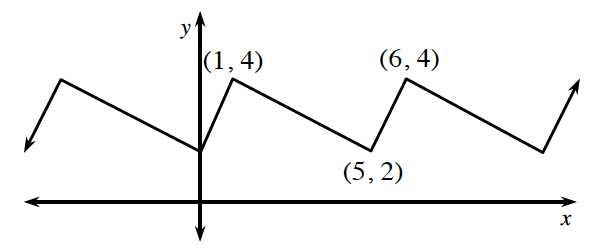### Home > PC3 > Chapter 2 > Lesson 2.2.3 > Problem2-83

2-83.

​​A periodic function is a function whose graph repeats itself identically, over and over, as it is followed from left to right. Periodic functions have “periodicity,” which simply means that they repeat at regular intervals. Many things you are familiar with also have this quality. Clocks have it, so do metronomes, and of course, periodicals.

The graph of $y=f(x)$ is a periodic function. Use it to complete parts (a) through (d) below.1. The period of a periodic function can be described as the smallest horizontal shift possible that preserves the function’s appearance. What is the period of $f$ ?

One maximum is at $x=1$. The next maximum is at $x=6$. The period of this function is the distance between these two points.

2. Even though the points are not shown on the graph, what are $f(-14)$ and $f(105)$? Explain how you determined these values.

$f(1)=f(6)=f(15)=\dots$
$f(5)=f(10)=\dots$

3. Is $f(2)$ equal to $f(50)$? Explain.

$f(2)=f(7)=\dots$

4. Use the idea of transformations of functions to write an equivalent expression for $f(x)$ in this situation.

Review the hints in parts (c) and (d).